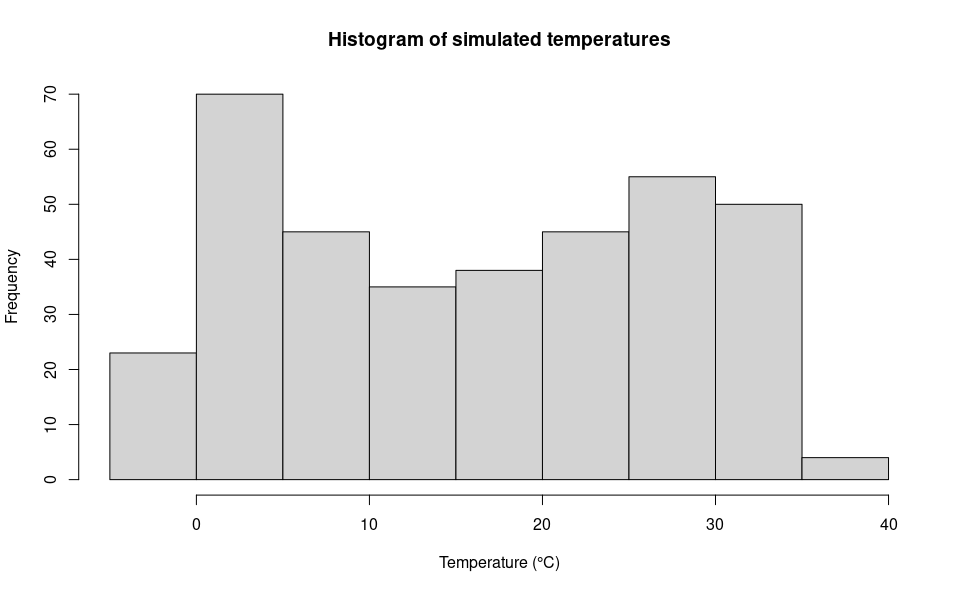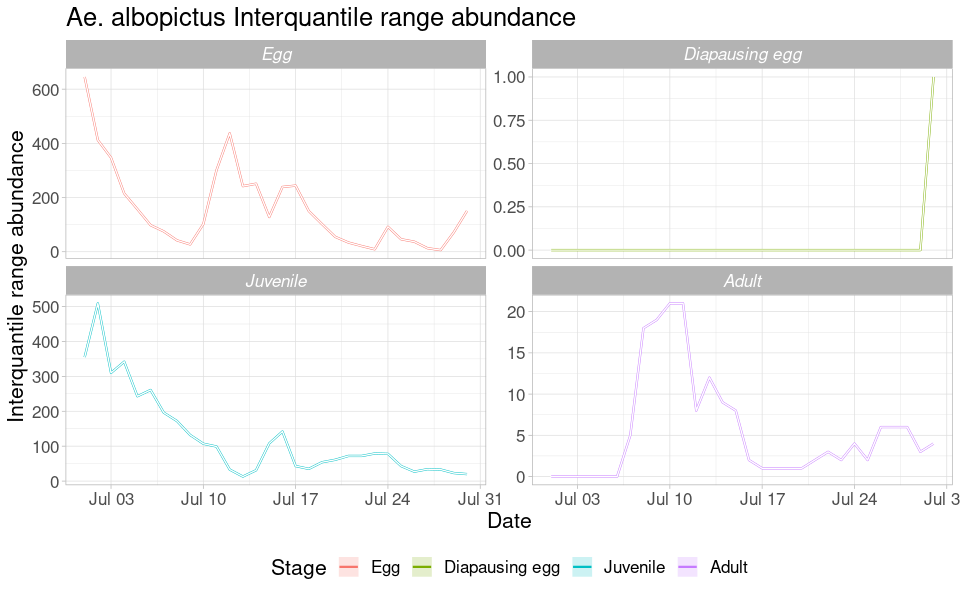# The punctual scale model

#### 2023-03-30

This tutorial explains step-by-step the main features of dynamAedes package, a unified modelling framework for invasive Aedes mosquitoes. Users can apply the stochastic, time-discrete and spatially-explicit population dynamical model initially developed in Da Re et al., (2021) for Aedes aegypti and then expanded for other three species: Ae. albopictus, Ae. japonicus and Ae. koreicus Da Re et al., (2022).

The model is driven by temperature, photoperiod and intra-specific larval competition and can be applied to three different spatial scales: punctual, local and regional. These spatial scales consider different degrees of spatial complexity and data availability, by accounting for both active and passive dispersal of the modelled mosquito species as well as for the heterogeneity of input temperature data.

We will describe the model applications for Ae. albopictus and for all spatial scales by using a simulated temperature dataset.

#Load packages
require(spatstat)
require(gstat)
require(parallel)
require(eesim)
require(dplyr)
require(geosphere)
require(ggplot2)
require(terra)
require(rgdal)
require(raster)
require(dynamAedes)

Sys.setlocale("LC_TIME", "en_GB.UTF-8")  
#  "en_GB.UTF-8"

# Punctual scale model

At punctual scale, the model only requires a weather station temperature time series provided as a numerical matrix (in degree Celsius). For the purpose of this tutorial, we simulate a 1-year long temperature time series.

## Simulate temperature data with seasonal trend

We first simulate a 1-year temperature time series with seasonal trend. For the time series we consider a mean value of 16°C and standard deviation of 2°C.

ndays = 365*1 #length of the time series in days
set.seed(123)
sim_temp <- create_sims(n_reps = 1,
n = ndays,
central = 16,
sd = 2,
exposure_type = "continuous",
exposure_trend = "cos1", exposure_amp = -1.0,
average_outcome = 12,
outcome_trend = "cos1",
outcome_amp = 0.8,
rr = 1.0055)

A visualisation of the distribution of temperature values and temporal trend.

hist(sim_temp[]$x, xlab="Temperature (°C)", main="Histogram of simulated temperatures")plot(sim_temp[]$date,

### Run the model

Running the model with the settings specified in this example takes about 2 minutes.

simout <- dynamAedes.m(species="albopictus",
scale="ws",
jhwv=habitat_liters,
temps.matrix=w,
startd=str,
endd=endr,
n.clusters=cl,
iter=it,
intro.eggs=ie,
compressed.output=TRUE,
lat=myLat,
long=myLon,
verbose=FALSE,
seeding=TRUE)
#
|
|                                                                      |   0%

## Analyse the results

We first explore the model output structure: the simout object is a nested list.

The first level corresponds to the number of model iterations

print(it)
#  1
print(length(simout))
#  1

The second level corresponds to the simulated days. So if we inspect the first iteration, we observe that the model has computed rlength(simout[]) days, since we have started the simulation on the 1st of July and ended on the 1st of August.

length(simout[])
#  30

The third level corresponds to the amount of individuals for each stage (rows). So if we inspect the 1st and the 15th day within the first iteration, we obtain a matrix having

dim(simout[][])
#  4 1
simout[][]
#              [,1]
# egg           645
# juvenile      355
# diapause_egg    0
simout[][]
#              [,1]
# egg           128
# juvenile      108
# diapause_egg    0

We can now use the auxiliary functions of the package to analyse the results.

### Derive probability of a successfull introduction at the end of the simulated period

First, we can retrieve the “probability of successful introduction”, computed as the proportion of model iterations that resulted in a viable mosquito population at a given date. In this case the results is 1, since we have only one iteration and the population is still viable at the end of the simulation.

psi(input_sim = simout, eval_date = 30)
#   Days_after_intro p_success      stage
# 1           Day 30         1 Population

### Derive abundance 95% CI for each life stage and in each day

We now compute the interquantile range abundance for all the stages of the simulated population using the function adci.

# Retrieve the maximum number of simulated days
dd <- max(sapply(simout, function(x) length(x)))

# Compute the abundance
outdf <- rbind(data.frame(cbind(
myStage = 'Egg')),
data.frame(cbind(
myStage='Juvenile')),
data.frame(cbind(
data.frame(cbind(
myStage='Diapausing egg'))
)

# Plot
outdf %>%
mutate(myStage=factor(myStage, levels= c('Egg', 'Diapausing egg', 'Juvenile', 'Adult')),
X25.=as.numeric(X25.),
X50.=as.numeric(X50.),
X75.=as.numeric(X75.),
Date=rep(seq.Date(as.Date(str), as.Date(endr)-2, by="day"),4)) %>%
ggplot( aes(x=Date, y=X50., group=factor(myStage),col=factor(myStage))) +
ggtitle("Ae. albopictus Interquantile range abundance")+
geom_line(linewidth=0.8)+
geom_ribbon(aes(ymin=X25.,ymax=X75.,fill=factor(myStage)),
col="white",
alpha=0.2,
outline.type="full")+
labs(x="Date", y="Interquantile range abundance", col="Stage", fill="Stage")+
facet_wrap(~myStage, scales = "free_y")+
theme_light()+
theme(legend.pos="bottom",
text = element_text(size=16) ,
strip.text = element_text(face = "italic"))